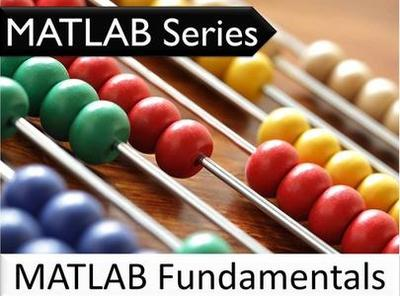MATLAB系列：MATLAB基础知识类别 ： 视频教程 格式 ： MP4
 订阅须知  订阅

### MATLAB系列：MATLAB基础知识

• MATLAB背景。本MATLAB系列中的第一个主题将向您介绍MATLAB。了解这种强大的编程语言的历史。
• MATLAB的好处。本MATLAB系列的第二个主题说明了使用MATLAB的好处，包括矩阵，工具箱，专用功能，精确的仿真配置文件和操作的可靠性。
• MATLAB用例。本MATLAB系列的第三个主题揭示了MATLAB的用途，包括使用线性代数，执行数值分析，建模，仿真，原型设计，开发应用程序以及提供探索和可视化功能。提供了MATLAB的实际应用程序，包括嵌入式系统，控制系统，数字信号处理（DSP），无线通信，物联网，机器人技术和深度学习。
• MATLAB案例研究。本MATLAB系列的第四个主题使用医学成像示例演示了MATLAB的功能，以进行数据可视化和分析。
• MATLAB系统。本MATLAB系列的第五个主题详细介绍了MATLAB系统，包括MATLAB语言，MATLAB环境，图形管理，数学库函数和MATLAB应用程序编程接口（API）。
• MATLAB功能。本MATLAB系列的第六个主题介绍了MATLAB的主要功能，包括数据元素和工具箱。
• MATLAB安装。本MATLAB系列的第7个主题向您展示如何安装MATLAB，以及创建和运行几个简短的脚本。